# Catch up on the latest AI articles# Can ChatGPT Help You Pass The University's Computer Science Department Exam!

3 main points
✔️ To evaluate ChatGPT's performance in computer science, a blind test was conducted in which students were asked to answer an actual university exam on ChatGPT
✔️ With an average student score of 23.9, ChatGPT-3.5 scored 20.5 and ChatGPT-4 scored 24, a high score. Recorded
✔️ Comparison of ChatGPT-3.5 and ChatGPT-4 performance and analysis of their limitations based on test scoring results

written by Sebastian BordtUlrike von Luxburg
(Submitted on 22 Mar 2023)

Subjects: Computation and Language(cs.CL); Computers and Society(cs.CY)code：The images used in this article are from the paper, the introductory slides, or were created based on them.

## Introduction

Large-scale language models are currently achieving remarkable results in a variety of multitudes and have been reported to have diverse problem-solving capabilities, including specific tasks such as coding and solving advanced mathematical problems.

However, while the capabilities of these language models have improved dramatically, there has been no research focused on developing new benchmarks to evaluate these models, plus there has been little systematic examination of ChatGPT's performance on university exams.

This paper focuses on the development of a new benchmark for evaluating large-scale language models, and describes a paper that examines the performance limitations of large-scale language models in the field of computer science by having ChatGPT answer questions from an actual university final exam and analyzing the results. The paper examines the performance limits of large-scale language models in computer science.

## Experimental Design

In this paper, ChatGPT was asked to answer questions on the final exam of the course " Algorithms and Data Strucutures," which is taken by students of the Department of Computer Science at the University of Tubingen, Germany, in order to measure the ability of large-scale language modeling in the field of computer science. Strucutures (Algorithms and Data Structures), a course for computer science students at the University of Tubingen, Germany.

The exam covers topics such as sorting algorithms, dynamic programming, and graph traversal (the process of accessing each vertex in a graph), and includes a variety of different types of questions , including choice questions, proof questions, graph drawing questions, and coding tests.

In addition, 200 students take this exam, and students submit handwritten answers on an exam paper, which is then graded by teaching assistants.

Since these tests were compiled in a single latex file and the figures in the tests were also generated by latex code consisting only of text, the questions were entered into ChatGPT as text and the output code was handwritten on the answer sheet as formulas, as shown in the figure below, to answer the questions andselect For the questions, the model was explicitly told which option was most appropriate.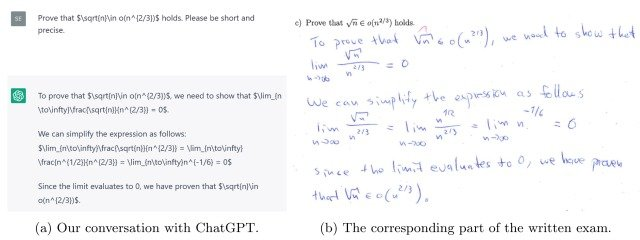Similarly, for the questions that required drawing a graph, we had ChatGPT output a latex command using tikz-graph, a library for latex graphing, and handwrote it on the answer sheet.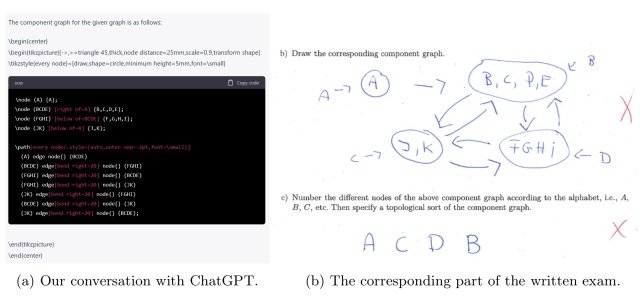In addition, since the content of the prompt is known to have a significant impact on ChatGPT output, we tried to enter the problem as simply as possible, as shown in the figure below, and did not use any chain of thought prompting, etc., which could increase the performance of the output. No prompt engineering was performed.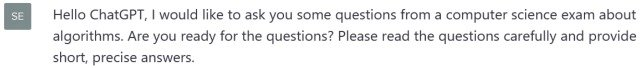The answer sheets generated by the ChatGPT output were then submitted along with the 200 student answer sheets and graded by the teaching assistants in the same way as a regular exam.

In addition, existing research on large-scale language models has discussed the possibility of bias in scoring the models' answers.

To address these issues, this study was conducted in the form of a blind test, and in order to obtain more accurate scoring results free of bias, teaching assistants were asked to score the study without being told about it beforehand.

## Results

The scoring results are shown in the figure below.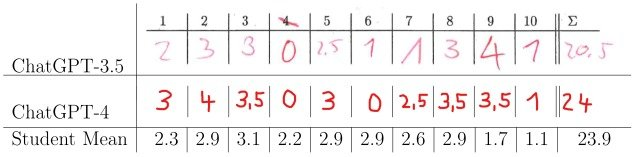The figure shows the average score of the 200 students who participated in this exam and the scores of the answers given by ChatGPT-3.5 and ChatGPT-4.

The experimental results show that the performance of both ChatGPT models exceeded the passing score (20 or more out of 40 points), and the performance of ChatGPT-4 exceeded the average score of the students.

### Analysis of solution results

Next, an analysis was performed on the ChatGPT-3.5 solution results.

What was particularly striking about the ChatGPT-3.5 answers was the very high percentage of correct answers to the proof questions, as shown in the figure below, and the output of answers that gave the impression that the model actually "understood" and solved these proof methods.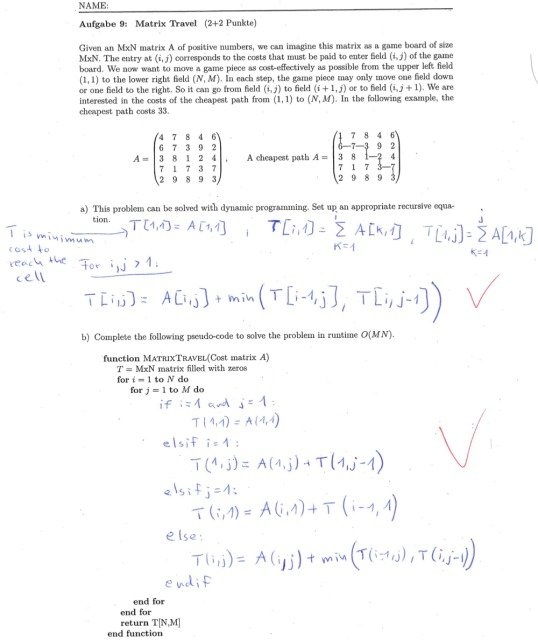The percentage of correct answers to the questions on dynamic programming in the figure is very poor among the students, and the good performance of ChatGPT on these questions is a major finding.

On the other hand, ChatGPT-3.5 was rarely able to solve graph drawing problems that illustrate the behavior of standard algorithms, such as the one shown below.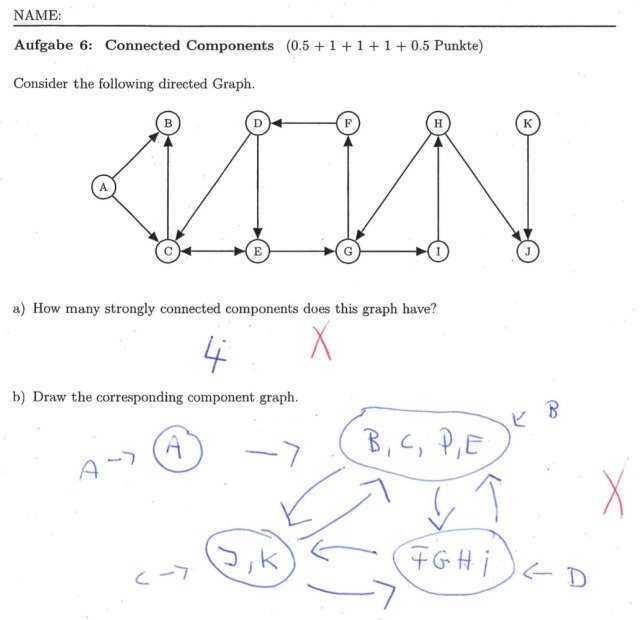Although these questions on algorithms are one of the easiest exercises in this exam, it was observed that the percentage of correct answers was low, for example, generating graphs with many loops.

On the other hand, students on the ChatGPT-4 were able to appropriately answer such graph drawing questions, and were also able to select appropriate correct answers for the more difficult choice questions that had poor correct answer rates among students.

These results confirm that ChatGPT-4 is a significant improvement over ChatGPT-3.5.

## summary

How was it? In this issue, we focused on the development of a new benchmark for evaluating large-scale language models, and discussed a paper that examines the performance limitations of large-scale language models in computer science by having ChatGPT answer questions from an actual university final exam and analyzing the results. The paper examined the performance limits of large-scale language models in the field of computer science.

In this experiment, both the ChatGPT-3.5 and ChatGPT-4 produced scores that exceeded the exam passing score, and in the ChatGPT-4, exceeded the average score of the 200 students who participated in the exam.

However, there is a lot of material on the Internet that contains information about the topics covered in this exam (sorting algorithms, dynamic programming, graph traversal, etc.) as well as sample answers to the exam, and it is likely that the ChatGPT training data will include many of these practice questions and sample answers. The ChatGPT training data may contain many of these practice questions and sample answers.

Therefore, the results of this experiment are insufficient to conclude that ChatGPT understands computer science, and more research will be needed in the future to verify the performance of these large-scale language models.

The details of the prompts used for ChatGPT in this experiment and the results of the test scoring can be found in this paper for those who are interested.

If you have any suggestions for improvement of the content of the article,